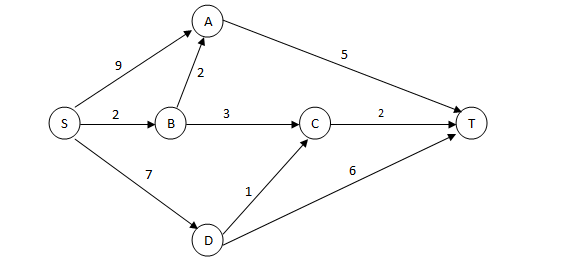### CAT 2006 Question Paper Question 41

Instructions

A significant amount of traffic flows from point S to point T in the one-way street network shown below. Points A, B, C, and D are junctions in the network, and the arrows mark the direction of traffic flow. The fuel cost in rupees for travelling along a street is indicated by the number adjacent to the arrow representing the street. –Motorists traveling from point S to point T would obviously take the route for which the total cost of traveling is the minimum. If two or more routes have the same least travel cost, then motorists are indifferent between them. Hence, the traffic gets evenly distributed among all the least cost routes.

The government can control the flow of traffic only by levying appropriate toll at each junction. For example, if a motorist takes the route S-A-T (using junction A alone), then the total cost of travel would be Rs 14 (i.e., Rs 9 + Rs 5) plus the toll charged at junction A.

Question 41

# If the government wants to ensure that all motorists travelling from S to T pay the same amount (fuel costs and toll combined) regardless of the route they choose and the street from B to C is under repairs (and hence unusable), then a feasible set of toll charged (in rupees) at junctions A, B, C, and D respectively to achieve this goal is:

Solution

Let the toll charged at junctions A, B, C, and D be a,b,c and d respectively. Then the so that equal amount is collected through all route we have, 9+a+5=2+b+2+a+5=10+d+c=13+d. Then from the options only option C satisfies the above equality. hence option C.

### View Video Solution

• All Quant CAT Formulas and shortcuts PDF
• 30+ CAT previous papers with solutions PDF

11 months, 2 weeks ago

Nice brilliant

##### Shree

2 months, 3 weeks ago

abcd-0,5,4,1 if this q is tita, am i right?

OR